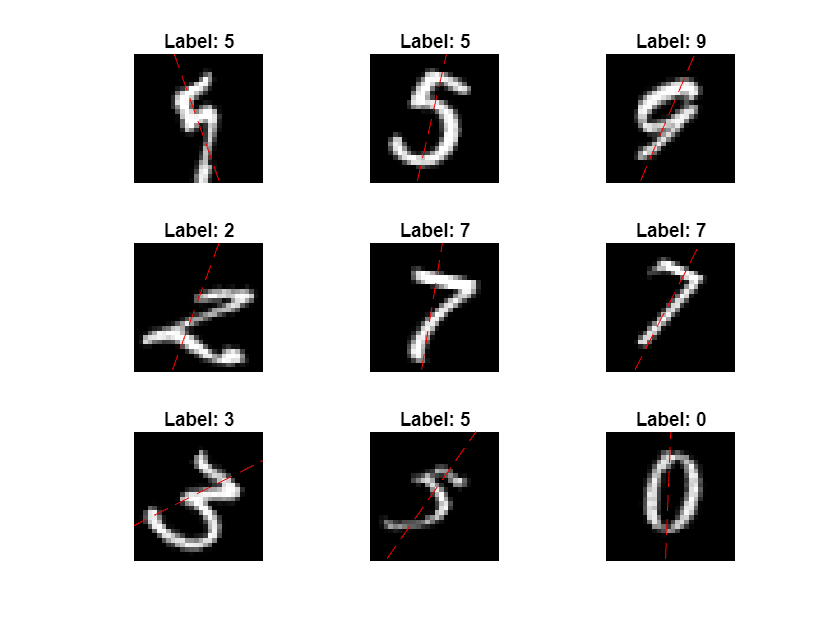## Make Predictions Using Model Function

This example shows how to make predictions using a model function by splitting data into mini-batches.

For large data sets, or when predicting on hardware with limited memory, make predictions by splitting the data into mini-batches. When making predictions with `SeriesNetwork` or `DAGNetwork` objects, the `predict` function automatically splits the input data into mini-batches. For model functions, you must split the data into mini-batches manually.

### Create Model Function and Load Parameters

Load the model parameters from the MAT file `digitsMIMO.mat`. The MAT file contains the model parameters in a struct named `parameters`, the model state in a struct named `state`, and the class names in `classNames`.

```s = load("digitsMIMO.mat"); parameters = s.parameters; state = s.state; classNames = s.classNames;```

The model function `model`, listed at the end of the example, defines the model given the model parameters and state.

Load the digits data for prediction.

```digitDatasetPath = fullfile(matlabroot,'toolbox','nnet','nndemos', ... 'nndatasets','DigitDataset'); imds = imageDatastore(digitDatasetPath, ... 'IncludeSubfolders',true, ... 'LabelSource','foldernames');```

### Make Predictions

Loop over the mini-batches of the test data and make predictions using a custom prediction loop.

For each mini-batch:

• Convert the data to `dlarray` objects with underlying type single and specify the dimension labels `'SSCB'` (spatial, spatial, channel, batch).

• Make predictions on a GPU if one is available. Using a GPU requires Parallel Computing Toolbox™ and a CUDA® enabled NVIDIA® GPU with compute capability 3.0 or higher.

• Make predictions by calling the model function with the `doTraining` option set to `false`.

• Determine the class labels by finding the maximum scores.

```miniBatchSize = 128; executionEnvironment = "auto"; doTraining = false; imds.ReadSize = miniBatchSize; numObservations = numel(imds.Files); Y1Pred = strings(1,numObservations); Y2Pred = zeros(1,numObservations); i = 1; % Loop over mini-batches. while hasdata(imds) % Read mini-batch of data. data = read(imds); X = cat(4,data{:}); % Normalize the images. X = single(X)/255; % Convert mini-batch of data to dlarray. dlX = dlarray(X,'SSCB'); % If making predictions on a GPU, then convert data to gpuArray. if (executionEnvironment == "auto" && canUseGPU) || executionEnvironment == "gpu" dlX = gpuArray(dlX); end % Make predictions using the predict function. [dlY1Pred,dlY2Pred] = model(dlX,parameters,doTraining,state); % Determine corresponding classes. [~,idxTop] = max(extractdata(dlY1Pred),[],1); idxMiniBatch = i:min((i+miniBatchSize-1),numObservations); Y1Pred(idxMiniBatch) = classNames(idxTop); Y2Pred(idxMiniBatch) = gather(extractdata(dlY2Pred)); i = i + miniBatchSize; end```

View some of the images with their predictions.

```idx = randperm(numel(imds.Files),9); figure for i = 1:9 subplot(3,3,i) I = imread(imds.Files{idx(i)}); imshow(I) hold on sz = size(I,1); offset = sz/2; thetaPred = Y2Pred(idx(i)); plot(offset*[1-tand(thetaPred) 1+tand(thetaPred)],[sz 0],'r--') hold off label = string(Y1Pred(idx(i))); title("Label: " + label) end```### Model Function

The function model takes the input data `dlX`, the model parameters `parameters`, the flag `doTraining` which specifies whether to model should return outputs for training or prediction, and the network state `state`. The network outputs the predictions for the labels, the predictions for the angles, and the updated network state.

```function [dlY1,dlY2,state] = model(dlX,parameters,doTraining,state) % Convolution W = parameters.conv1.Weights; B = parameters.conv1.Bias; dlY = dlconv(dlX,W,B,'Padding',2); % Batch normalization, ReLU Offset = parameters.batchnorm1.Offset; Scale = parameters.batchnorm1.Scale; trainedMean = state.batchnorm1.TrainedMean; trainedVariance = state.batchnorm1.TrainedVariance; if doTraining [dlY,trainedMean,trainedVariance] = batchnorm(dlY,Offset,Scale,trainedMean,trainedVariance); % Update state state.batchnorm1.TrainedMean = trainedMean; state.batchnorm1.TrainedVariance = trainedVariance; else dlY = batchnorm(dlY,Offset,Scale,trainedMean,trainedVariance); end dlY = relu(dlY); % Convolution, batch normalization (Skip connection) W = parameters.convSkip.Weights; B = parameters.convSkip.Bias; dlYSkip = dlconv(dlY,W,B,'Stride',2); Offset = parameters.batchnormSkip.Offset; Scale = parameters.batchnormSkip.Scale; trainedMean = state.batchnormSkip.TrainedMean; trainedVariance = state.batchnormSkip.TrainedVariance; if doTraining [dlYSkip,trainedMean,trainedVariance] = batchnorm(dlYSkip,Offset,Scale,trainedMean,trainedVariance); % Update state state.batchnormSkip.TrainedMean = trainedMean; state.batchnormSkip.TrainedVariance = trainedVariance; else dlYSkip = batchnorm(dlYSkip,Offset,Scale,trainedMean,trainedVariance); end % Convolution W = parameters.conv2.Weights; B = parameters.conv2.Bias; dlY = dlconv(dlY,W,B,'Padding',1,'Stride',2); % Batch normalization, ReLU Offset = parameters.batchnorm2.Offset; Scale = parameters.batchnorm2.Scale; trainedMean = state.batchnorm2.TrainedMean; trainedVariance = state.batchnorm2.TrainedVariance; if doTraining [dlY,trainedMean,trainedVariance] = batchnorm(dlY,Offset,Scale,trainedMean,trainedVariance); % Update state state.batchnorm2.TrainedMean = trainedMean; state.batchnorm2.TrainedVariance = trainedVariance; else dlY = batchnorm(dlY,Offset,Scale,trainedMean,trainedVariance); end dlY = relu(dlY); % Convolution W = parameters.conv3.Weights; B = parameters.conv3.Bias; dlY = dlconv(dlY,W,B,'Padding',1); % Batch normalization Offset = parameters.batchnorm3.Offset; Scale = parameters.batchnorm3.Scale; trainedMean = state.batchnorm3.TrainedMean; trainedVariance = state.batchnorm3.TrainedVariance; if doTraining [dlY,trainedMean,trainedVariance] = batchnorm(dlY,Offset,Scale,trainedMean,trainedVariance); % Update state state.batchnorm3.TrainedMean = trainedMean; state.batchnorm3.TrainedVariance = trainedVariance; else dlY = batchnorm(dlY,Offset,Scale,trainedMean,trainedVariance); end % Addition, ReLU dlY = dlYSkip + dlY; dlY = relu(dlY); % Fully connect (angles) W = parameters.fc1.Weights; B = parameters.fc1.Bias; dlY2 = fullyconnect(dlY,W,B); % Fully connect, softmax (labels) W = parameters.fc2.Weights; B = parameters.fc2.Bias; dlY1 = fullyconnect(dlY,W,B); dlY1 = softmax(dlY1); end```